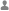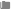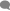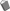## php数组合并array_merge与+的区别Veris分类：PHP0条评论已被围观 673

rray_merge()是PHP语言中的一个函数，作用是将两个或多个数组的单元合并起来，一个数组中的值附加在前一个数组的后面。返回作为结果的数组。

array_merge(array1[,array2[,array3...]])

 参数 描述 array1 必需。输入的第一个数组。 array2 必需。输入的第二个数组。 array3 可选。可指定的多个输入数组。

```<?php
\$a1=array("a"=>"Horse","b"=>"Dog");
\$a2=array("c"=>"Cow","b"=>"Cat");
print_r(array_merge(\$a1,\$a2));
?>```

`Array ( [a] => Horse [b] => Cat [c] => Cow )`

```<?php
\$a = array(3 => "Horse", 4 => "Dog");
print_r(array_merge(\$a));
?>```

`Array (  => Horse  => Dog )`

```<?php
\$a1=array("a"=>"Horse","b"=>"Dog");
\$a2=array("a"=>"Cow","b"=>"Cat");
print_r(array_merge(\$a1,\$a2));
?>```

`Array ( [a] => Cow [b] => Cat )`

“＋”运算符和array_merge():array array_merge ( array array1, array array2 [, array ...] ) 都可以合并多个数组，但使用过程中有一点小区别。

array_merge在参考手册中的说明如下：

array_merge()将两个或多个数组的单元合并起来，一个数组中的值附加在前一个数组的后面。返回作为结果的数组。

1.数组键名为数字键名时，要合并的两个数组中有同名数字KEY的时候，使用array_merge()不会覆盖掉原来的值，而使用“＋”合并数组则会把最先出现的值作为最终结果返回，而把后面的数组拥有相同键名的那些值“抛弃”掉（注意：不是覆盖而是保留最先出现的那个值）。例子：

```<?php
\$array1 = array(1=>'0');
\$array2 = array(1=> "data");
\$result1 = \$array2 + \$array1;/*结果为\$array2的值*/
print_r(\$result);
\$result = \$array1 + \$array2 ;/*结果为\$array1的值*/
print_r(\$result);
\$result3 = array_merge(\$array2,\$array1);/*结果为\$array2和\$array1的值，键名被重新分配*/
print_r(\$result3);
\$result4 = array_merge(\$array1,\$array2);/*结果为\$array1和\$array2的值，键名被重新分配*/
print_r(\$result4);
?>```

```Array (  => data )
Array (  => 0 )
Array (  => data  => 0 )
Array (  => 0  => data )```

2.当相同数组键名为字符时，“＋”运算符与键名为数字时一样，但array_merge()此时会覆盖掉前面相同键名的值。例子：

```<?php
\$array1 = array('asd'=>'0');
\$array2 = array('asd' => "data");
\$result1 = \$array2 + \$array1;/*结果为\$array2的值*/
print_r(\$result);
\$result = \$array1 + \$array2 ;/*结果为\$array1的值*/
print_r(\$result);
\$result3 = array_merge(\$array2,\$array1);/*结果为\$array1*/
print_r(\$result3);
\$result4 = array_merge(\$array1,\$array2);/*结果为\$array2*/
print_r(\$result4);
?>```

```Array ( [asd] => data )
Array ( [asd] => 0 )
Array ( [asd] => 0 )
Array ( [asd] => data )```﻿